# 揭秘在召唤师峡谷中移动路径选择逻辑？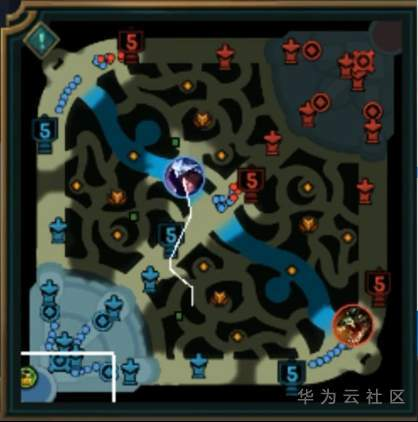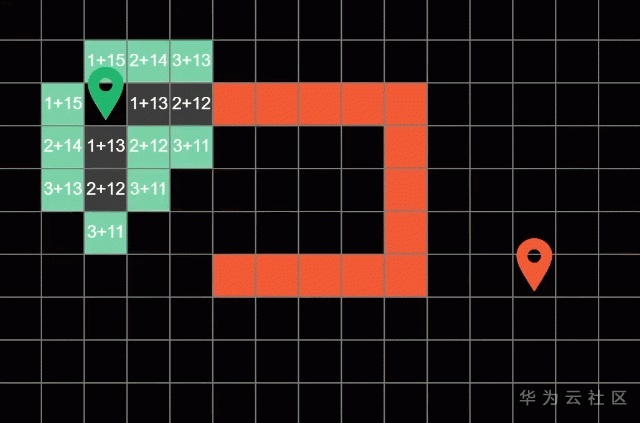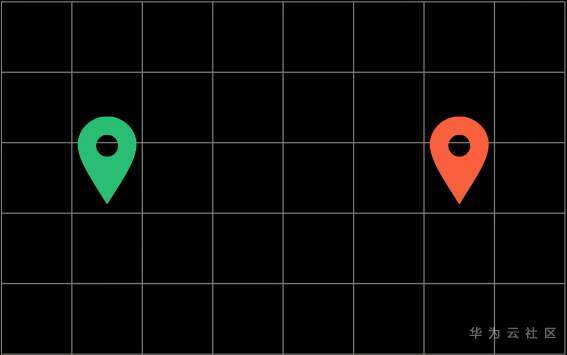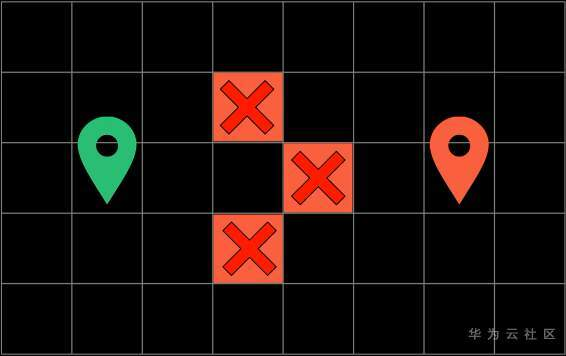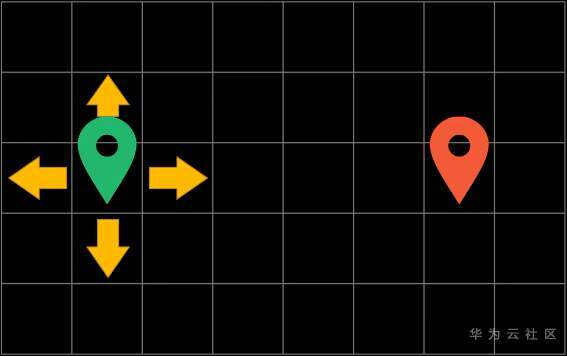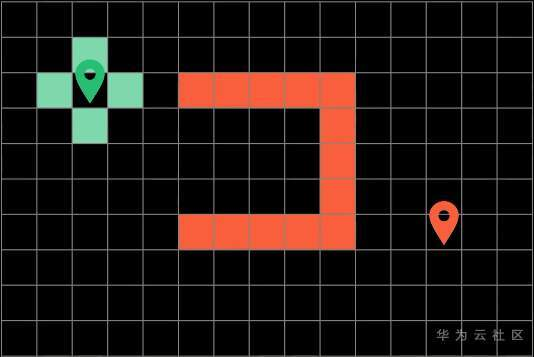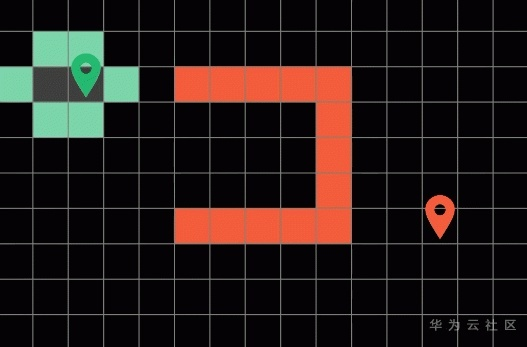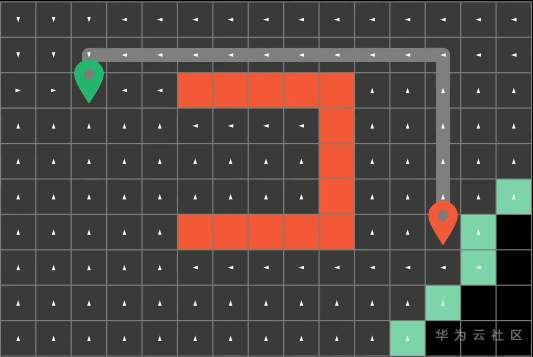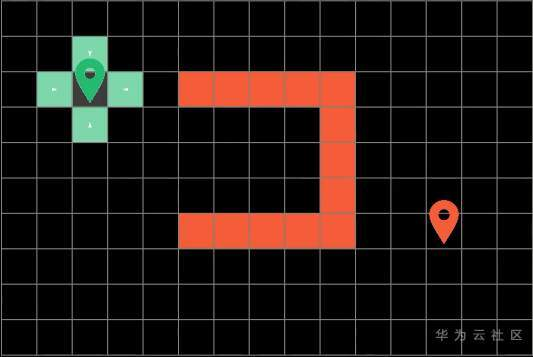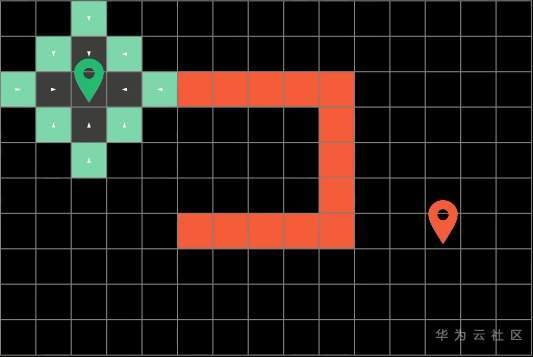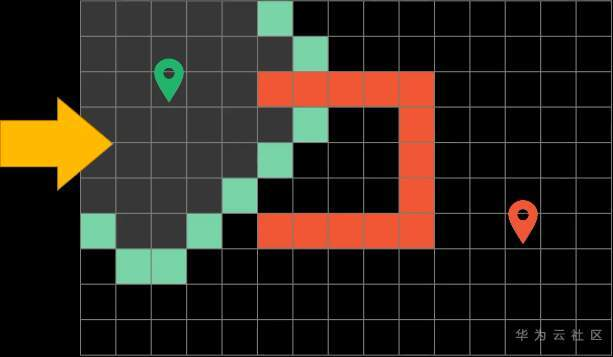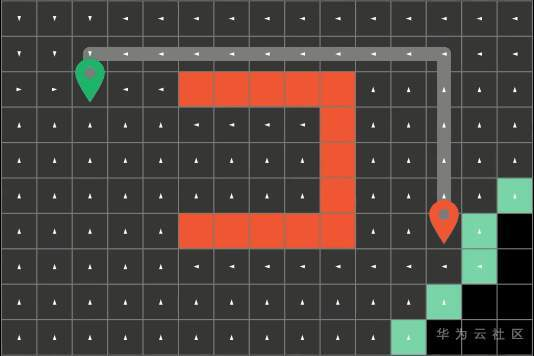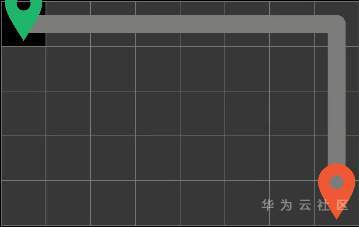## A* Search Algorithm

”A*搜索算法“也被叫做“启发式搜索”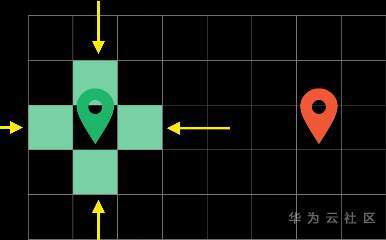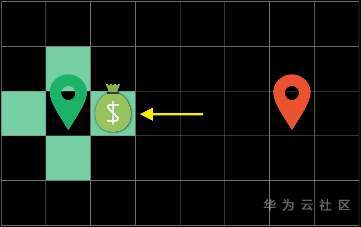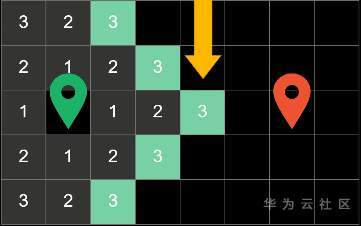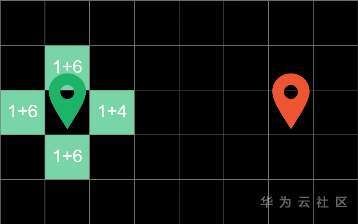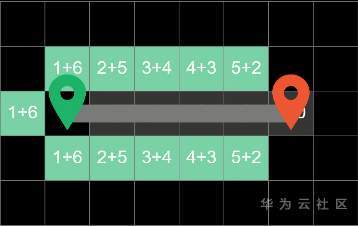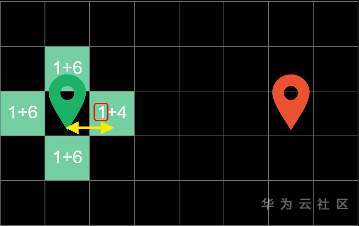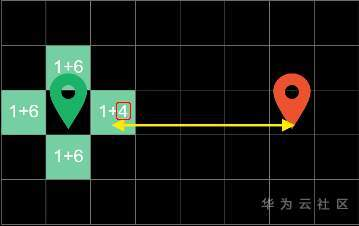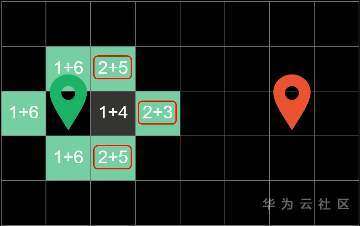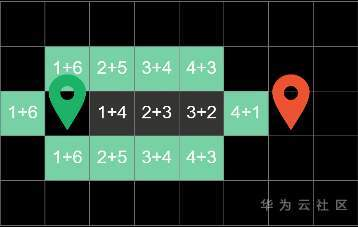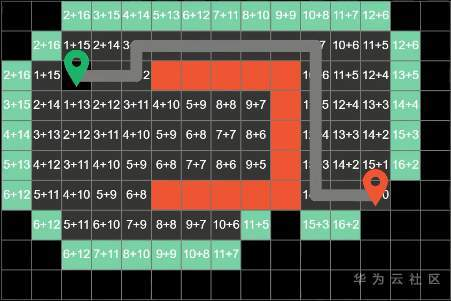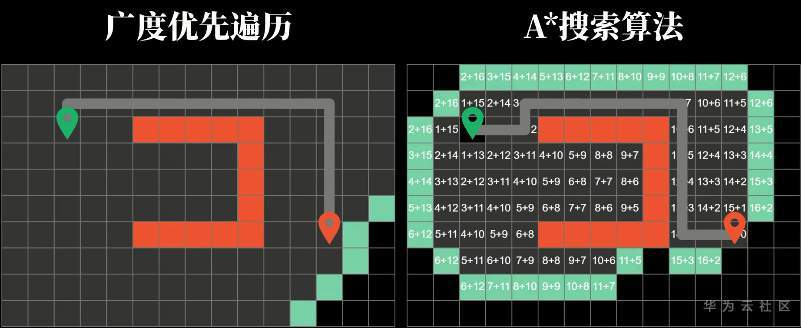def heuristic(a, b): #Manhattan Distance    (x1, y1) = a    (x2, y2) = b    return abs(x1 - x2) + abs(y1 - y2)def a_star_search(graph, start, goal): frontier = PriorityQueue()    frontier.put(start, 0)    came_from = {}    cost_so_far = {}    came_from[start] = None    cost_so_far[start] = 0        while not frontier.empty():        current = frontier.get()                if current = goal:            break                    for next in graph.neighbors(current):            new_cost = cost_so_far[current] + graph.cost(current, next)            if next not in cost_so_far or new_cost < cost_so_far[next]:                cost_so_far[next] = new_cost                priority = new_cost + heuristic(goal, next)                frontier.put(next, priority)                came_from[next] = current                    return came_from, cost_so_far

frontier = PriorityQueue()

PriorityQueue 代表它是一个优先队列，就是说它能够用“代价”自动排序，并每次取出”代价“最低的方块

frontier.put(start, 0)

came_from = {}

cost_so_far = {}

came_from[start] = Nonecost_so_far[start] = 0

while not frontier.empty(): current = frontier.get()

if current = goal: break

for next in graph.neighbors(current):

new_cost = cost_so_far[current] + graph.cost(current, next)

if next not in cost_so_far or new_cost < cost_so_far[next]:

frontier.put(next, priority)

priority = new_cost + heuristic(goal, next)

def heuristic(a, b):     (x1, y1) = a     (x2, y2) = b     return abs(x1 - x2) + abs(y1 - y2)

## 拓展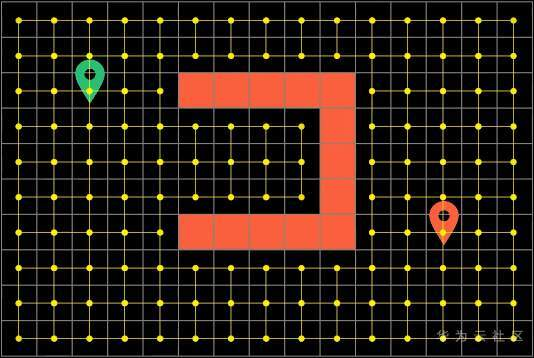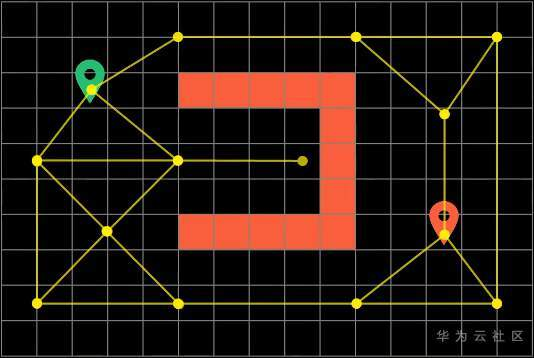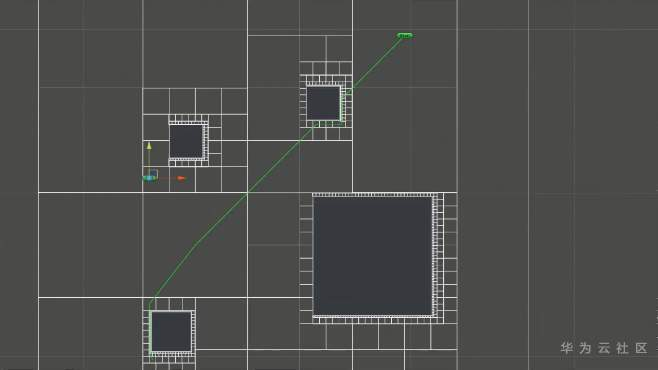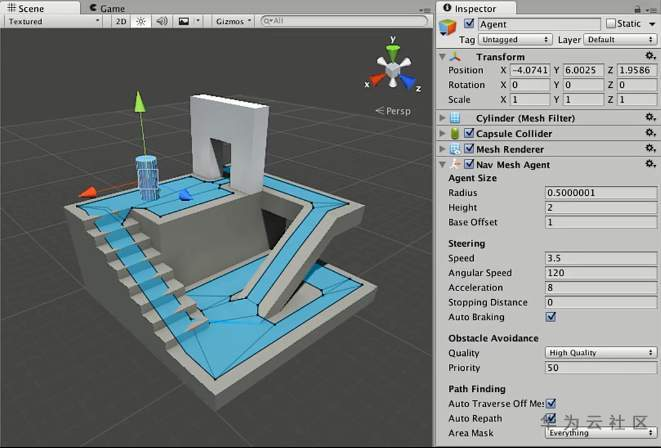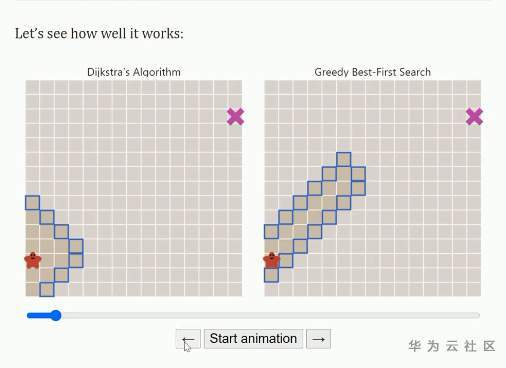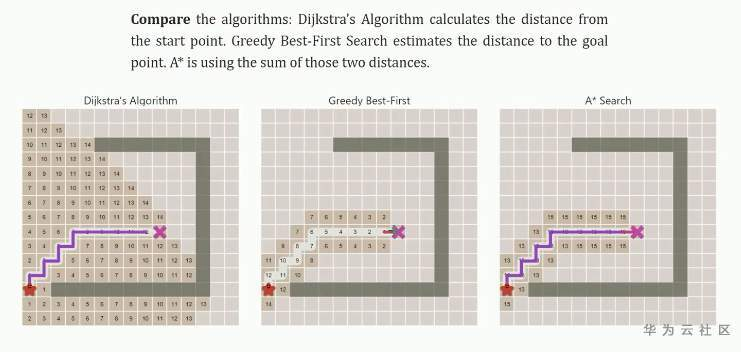周小镜. 基于改进 A 算法的游戏地图寻径的研究[D].西南大学,2010. https://www.redblobgames.com/pathfinding/a-star/introduction.html https://en.wikipedia.org/wiki/A_search_algorithm## 评论